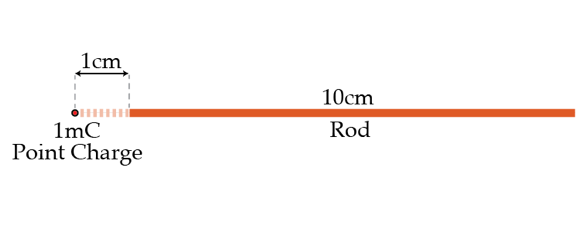# A rod near a point charge

A point charge of magnitude 1 mC is brought to a distance of 1 cm from the end of a horizontal, one-dimensional uniformly charged non-conducting rod of length 10 cm. The point charge is on the axis of the rod. If the net horizontal force on the rod is 10 N, what is the charge of the rod in nano-Coulombs?Details and assumptions

• You may take k to be $9 \times 10^9~\mbox{Nm}^2/\mbox{C}^2$.
×